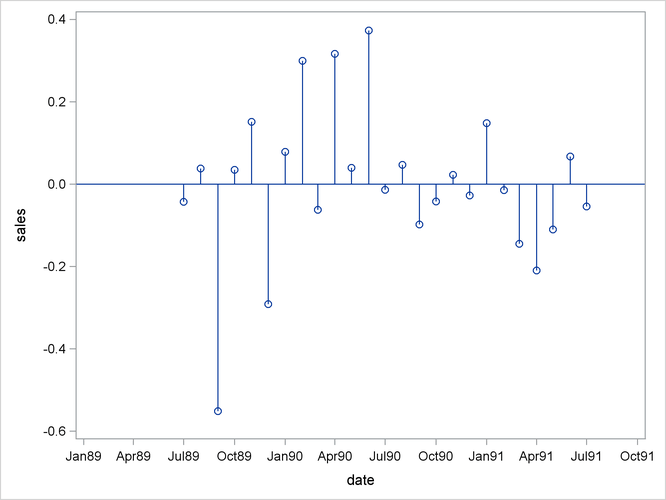# The FORECAST Procedure

### Plotting Residuals

You can plot the residuals from the forecasting model by using PROC SGPLOT and a WHERE statement.

1. Use the OUTRESID option or the OUTALL option in the PROC FORECAST statement to include the residuals in the output data set.

2. Use a WHERE statement to specify the observation type of ’RESIDUAL’ in the PROC GPLOT code.

The following statements add the OUTRESID option to the preceding example and plot the residuals:

```proc forecast data=past interval=month lead=10
out=pred outfull outresid;
id date;
var sales;
run;

proc sgplot data=pred;
where _type_='RESIDUAL';
needle x=date y=sales / markers;
xaxis values=('1jan89'd to '1oct91'd by qtr);
run;
```

The plot of residuals is shown in Figure 16.5.

Figure 16.5: Plot of Residuals